# Run a Function or a Loop only Once in PythonLast updated: Feb 20, 2023
4 min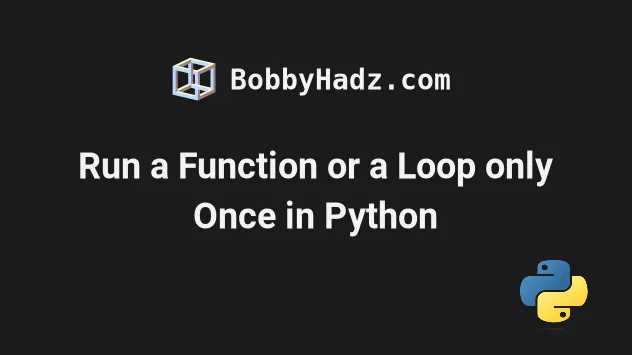## #Run a Function only Once in Python

To run a function only once:

1. Declare a global variable and initialize it to `False`.
2. Change the value of the global variable to `True` in the function.
3. Only run the code in the function if the global variable is set to `False`.
main.py
```Copied!```sum_has_run = False

def sum(a, b):
global sum_has_run

if sum_has_run:
return

sum_has_run = True

return a + b

print(sum(100, 100))  # 👉️ 200

print(sum(100, 100))  # 👉️ None
``````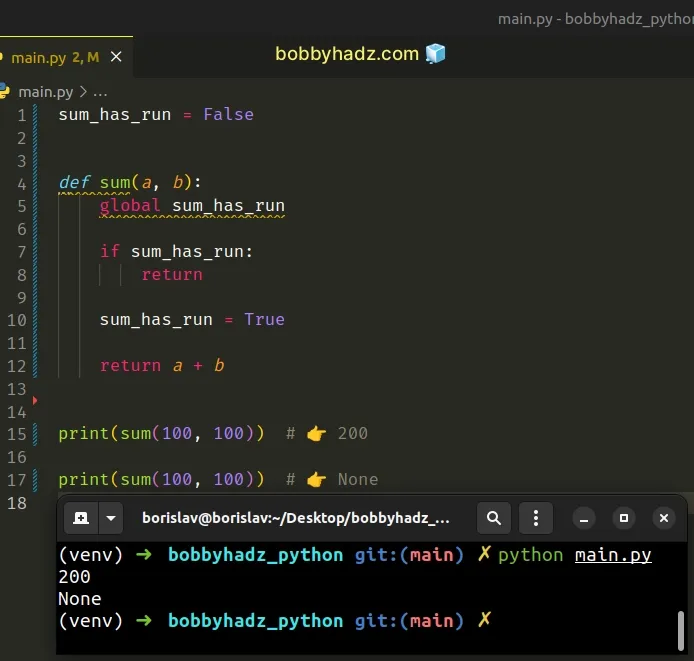The first example uses a global variable to run a function only once.

We initialize the global variable to `False` and set its value to `True` in the function.

If the value of the global variable is equal to `True`, we return from the function straight away.

main.py
```Copied!```sum_has_run = False

def sum(a, b):
global sum_has_run

if sum_has_run:
return

sum_has_run = True

return a + b

print(sum(100, 100))  # 👉️ 200

print(sum(100, 100))  # 👉️ None
``````
If the value of the global variable is not equal to `True`, we set its value to `True` and run the function.

This only happens the first time the function is invoked.

Once the global variable is set to `True`, the function returns None without running any code.

An alternative approach is to use an attribute on the function.

## #Run a Function only Once using an attribute

This is a three-step process:

1. Set a `has_run` attribute on the function to `True` the first time it runs.
2. Each time the function is called, check if the `has_run` attribute is `True` and return straight away.
3. The code in the function will only run the first time.
main.py
```Copied!```def sum(a, b):
if getattr(sum, 'has_run', False):
return

sum.has_run = True

return a + b

print(sum(100, 100))  # 👉️ 200

print(sum(100, 100))  # 👉️ None
``````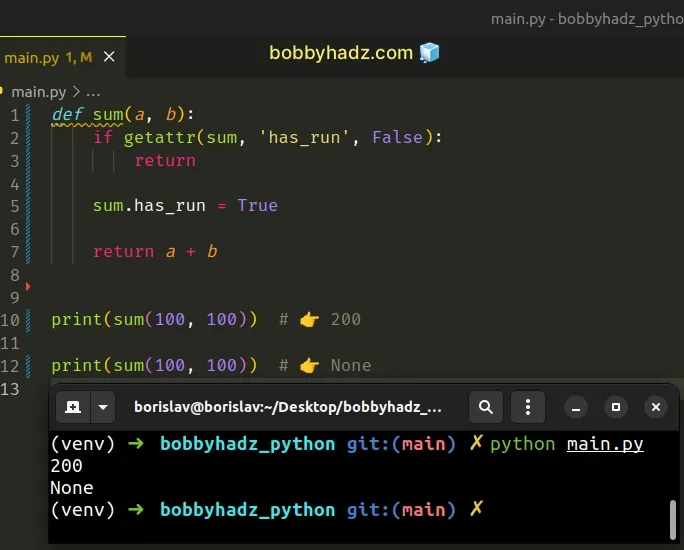We set the `has_run` attribute to `True` the first time the function is called.

The getattr function returns the value of the provided attribute of the object.

The function takes the object, the name of the attribute and a default value for when the attribute doesn't exist on the object as parameters.

If the `has_run` attribute is not set on the function, we return a default value of `False`.

We check for the value of the `has_run` attribute each time the function runs and if its value is set to `True`, we return from the function straight away.

If the `has_run` attribute is not set, the code in the function runs and sets the attribute to `True`.

The code in the function is only run once.

## #Run a Loop only Once in Python

You can run a loop only once by using the `range()` class with a `stop` value of `1`.

main.py
```Copied!```for i in range(1):
print('Loop is only run once')
``````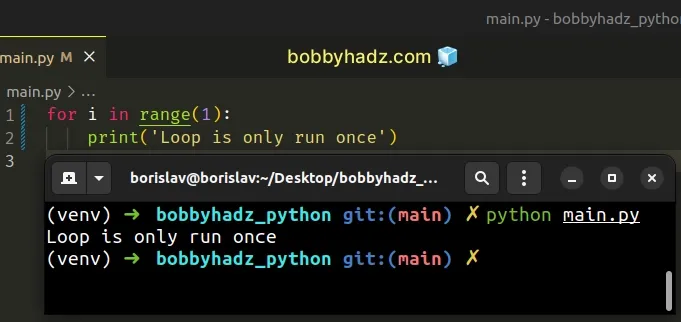The range class is commonly used for looping a specific number of times in `for` loops and takes the following arguments:

NameDescription
`start`An integer representing the start of the range (defaults to `0`)
`stop`Go up to, but not including the provided integer
`step`Range will consist of every N numbers from `start` to `stop` (defaults to `1`)

If you only pass a single argument to the `range()` constructor, it is considered to be the value for the `stop` parameter.

main.py
```Copied!```for n in range(1):
print(n) # 👉️ 0

result = list(range(1))

# 👇️ 
print(result)
``````

The `for` loop in the example only runs once.

## #Run a Loop only Once using a while True loop

You can also use a `while True` loop to only run a loop once.

main.py
```Copied!```while True:
print('Loop is only run once')
break
``````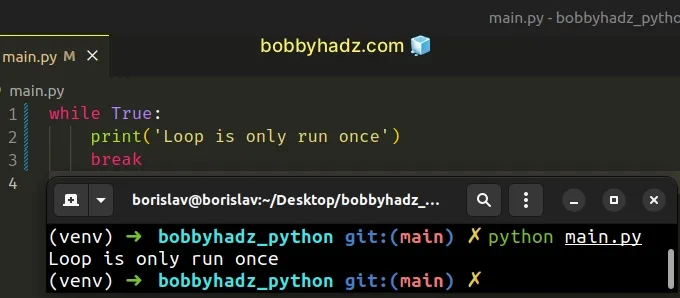A `while True` loop can only be stopped by using the `break` statement.

The break statement breaks out of the innermost enclosing `for` or `while` loop.

## #Run a Loop only Once using a break statement

You can also use the `break` statement to run a loop only once when iterating over a sequence.

main.py
```Copied!```a_list = ['bobby', 'hadz', 'com']

for item in a_list:
print(item) # 👉️ bobby
break
``````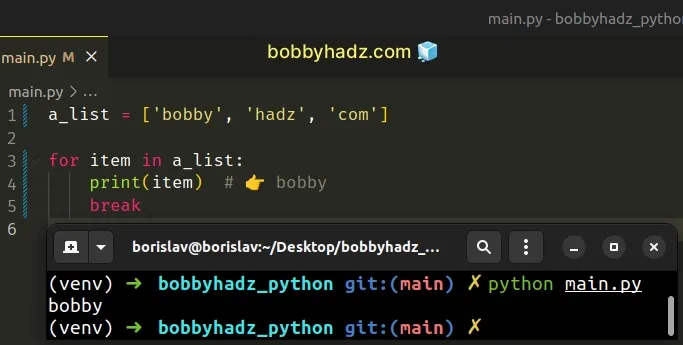The loop only prints the first item of the list and exits.

## #Run a Loop only Once using a boolean variable

You can also use a boolean variable to run a loop only once.

main.py
```Copied!```a_list = ['bobby', 'hadz', 'com']

has_run = False

for item in a_list:
if has_run:
break

has_run = True
print(item) # 👉️ bobby
``````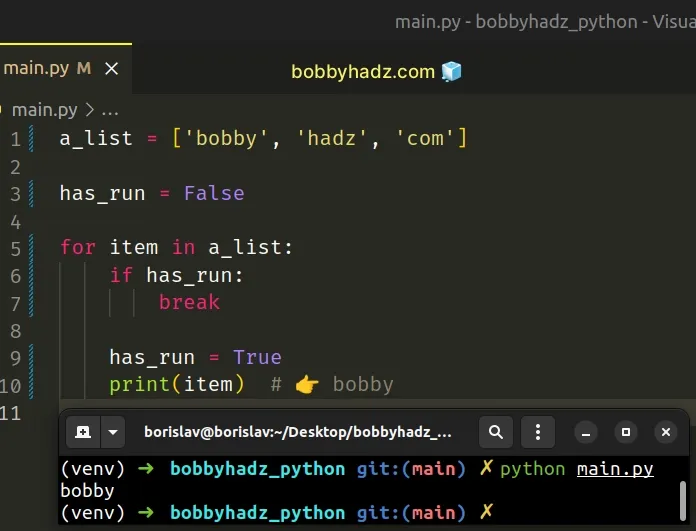We initialized the `has_run` variable to `False`.

The first time the `for` loop runs, we set the variable to `True`.

The next time the loop runs, the `if` condition is met and we exit out of the loop.

I've also written an article on how to call a function N times.

If you need to call a function by a string name, click on the link and follow the instructions.